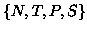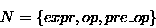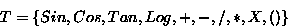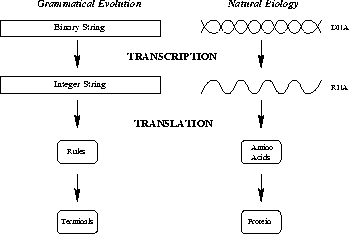Next: The Problem Spaces Up: Grammatical Evolution: A Steady Previous: Introduction

# Grammatical Evolution

Grammatical Evolution codes a set of pseudo random numbers on a chromosome which consists of a variable number of 8 bit binary genes. These numbers are used to select an appropriate rule from a Backus Naur Form grammar definition, an example of which is given below. A BNF grammar consists of the tuple, where N is the set of non-terminals, T, the set of terminals, P, a set of production rules that map the elements of N to T, and S is a start symbol which is a member of N. The non terminals of the grammar are then mapped onto the terminals of the grammar by continuously applying the rules dictated by the gene values. On completion of the mapping process the final code produced consists of these terminals.S = <expr>

And P can be represented as:

(1) <expr> ::= <expr> <op> <expr>     (A)
| ( <expr> <op> <expr> ) (B)
| <pre-op> ( <expr> )    (C)
| <var>                  (D)

(2) <op> ::= + (A)
| - (B)
| / (C)
| * (D)

(3) <pre-op> ::= Sin (A)
| Cos (B)
| Tan (C)
| Log (D)

(4) <var> ::= X


Consider rule #1 from the above example:

(1) <expr> ::= <expr> <op> <expr>
| ( <expr> <op> <expr> )
| <pre-op> ( <expr> )
| <var>


In this case, the non-terminal can produce one of four different results, to decide which one to use our system takes the next available random number from the chromosome and, in this case gets the modulus four of the number to decide which production to take . Each time a decision has to be made, another pseudo random number is read from the chromosome, and in this way, the system traverses the chromosome.

In a manner similar to natural biology [Elseth 95] the genes in GE are ultimately operated on to produce a phenotype, or in our sense, a program. The phenotype is produced as a result of a mapping process occurs which we liken to the process of gene transcription, followed by translation to a protein, see Figure 1.

Gene transcription is the process of converting a strand of DNA into a strand of RNA. The RNA then carries the encoded instructions of the genes to the machinery of the cell which can synthesize proteins. Translation is the mapping of the RNA onto amino acids. Put simply, the RNA is comprised of four different types of bases, groups of three bases, known as a codon, specify a particular amino acid. The amino acids are thus joined together in the order specified by the RNA to produce a protein. These proteins can act either independently or in conjunction with other proteins to produce a phenotype. To complete the analogy with GE then, the transcription process would be equivalent to the conversion of the binary string data structure into a string of integers which is brought from the Genetic Algorithm into the machinery of GE. The integers are then used in the translation process, that is the selection of rules in the BNF definition that result in the mapping of non-terminals to terminals. The production rules, equivalent to amino acids, combine to produce terminals, or proteins. It is the proteins that make up the final program.

In GE it is possible for individuals to run out of genes during the mapping process, and in this case there are two alternatives. The first is to declare the individual invalid and punish them with a suitably harsh fitness value; the alternative is to wrap the individual, and reuse the genes. This is quite an unusual approach in EAs, as it is entirely possible for certain genes to be used two or more times. What is crucial, however, is that each time a particular individual is mapped from its genotype to its phenotype, the same output is generated. This is because the same choices are made each time.To complete the BNF definition for a C function, we need to include the following rules with the earlier definition:

<func> ::= <header>

<header> ::= float symb(float X) { <body> }

<body> ::= <declarations><code><return>

<declarations> ::= float a;

<code> ::= a = <expr>;

<return> ::= return (a);


Notice that this function is limited to a single line of code, returns a float, and is passed a single float value X as a parameter. However, this is because of the nature of the problems tackled here, the system could easily generate functions which use several lines of code and a different parameter set by simply modifying the BNF grammar definition.Next: The Problem Spaces Up: Grammatical Evolution: A Steady Previous: Introduction
root
1998-10-02